## Dot product test

We define an adjoint when we write a program that computes one. In an abstract logical mathematical sense, however, every adjoint is defined by a dot product test. This abstract definition gives us no clues how to code our program. After we have finished coding, however, this abstract definition (which is actually a test) has considerable value to us.

Conceptually, the idea of matrix transposition is simply aij'=aji. In practice, however, we often encounter matrices far too large to fit in the memory of any computer. Sometimes it is also not obvious how to formulate the process at hand as a matrix multiplication. (Examples are differential equations and fast Fourier transforms.) What we find in practice is that an application and its adjoint amounts to two subroutines. The first subroutine amounts to the matrix multiplication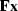.The adjoint subroutine computes,where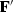is the conjugate-transpose matrix. Most methods of solving inverse problems will fail if the programmer provides an inconsistent pair of subroutines forand.The dot product test described next is a simple test for verifying that the two subroutines really are adjoint to each other.

The matrix expressionmay be written with parentheses as either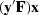or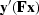.Mathematicians call this the associative'' property. If you write matrix multiplication using summation symbols, you will notice that putting parentheses around matrices simply amounts to reordering the sequence of computations. But we soon get a very useful result. Programs for some linear operators are far from obvious, for example causint(). Now we build a useful test for it.(16) (17)
For the dot-product test, load the vectorsand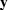with random numbers. Compute the vector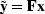using your program for,and compute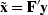using your program for.Inserting these into equation (17) gives you two scalars that should be equal.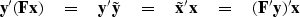(18)
The left and right sides of this equation will be computationally equal only if the program doingis indeed adjoint to the program doing(unless the random numbers do something miraculous). Note that the vectorsandare generally of different lengths.

Of course passing the dot product test does not prove that a computer code is correct, but if the test fails we know the code is incorrect. More information about adjoint operators, and much more information about inverse operators is found in my other books, Earth Soundings Analysis: Processing versus inversion (PVI) and Geophysical Estimation by Example (GEE).